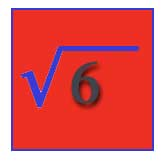# What Are Squares And Square Roots?The mathematical term 'square' comes from the two-dimensional shape of the same name. A square shape has the two dimensions of length and width, both exactly the same and at angles of 90 to each other. It is also perfectly flat. Put another way, a square is just as wide as it is long. The mathematical square of a number comes from the shape of a square by the number of standard-sized squares that it contains. For example, a square that measures 9 centimeters on a side contains 81 smaller squares that are each 1 centimeter on a side. This is easy to demonstrate by making a drawing of the square on a piece of graph paper that has been ruled into 1 centimeter squares. Draw a 9 centimeter square and count the smaller squares that it contains. There will be 81 of them.

That number was obtained by finding the area of the four-sided shape, multiplying the length of the figure by its width. That is, by multiplying one number by another number. For a square, the length and width are equal. Finding the area of a square therefore involves multiplying a number by itself. This brings us to the general definition of the square of a number. The square of any number is that number multiplied by itself.

For example, 81 is the square of 9 because you have to multiply 9 by itself (9) to get 81. The number that gets multiplied by itself to make the square value is called the root value of that square, or the square root. This is the basis of the general definition of a square root. The square root of a number is whatever number must be multiplied by itself ('squared') to get the original number. For example, 3 is the square root of 9, because 3 must be multiplied by 3 (itself) to get 9. These relationships are true for any number.

Richard M J Renneboog, MSRichard M. J. Renneboog is an independent private technical consultant and writer in both chemical and computer applications. Endeavors have included preparation of scripts for instructional and promotional video, corporate website design, curriculum development for training in advanced composites technology, and development.

### Science Articles: